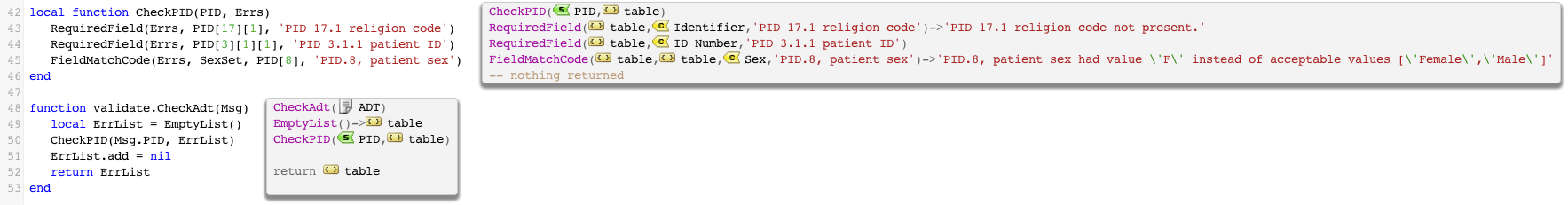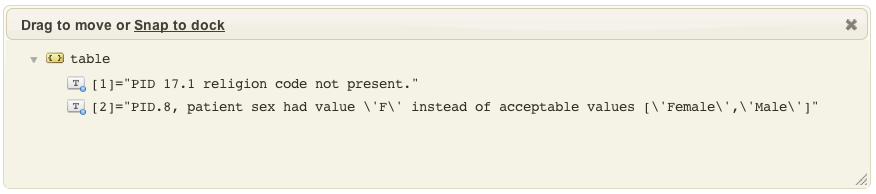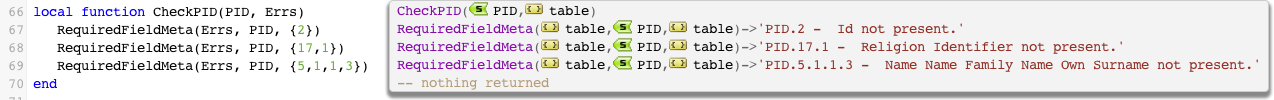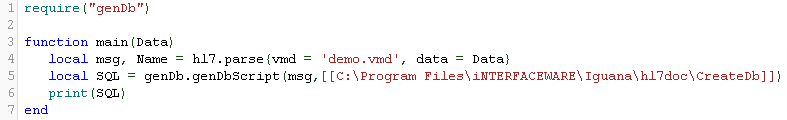# HL7 Conformance

This article was originally written for Iguana 5 and contains version 5 screenshots, and may contain out of date references.

Using the validate.lua module.

This is an area which is becoming more and more important as more data is exchanged between different health organizations. i.e. HIE (Health Information Exchange). If your organization can offer interfaces to your trading partners that are very rich and informative in telling what is wrong with the format of HL7 they are feeding you, then it can save an awful lot of time in creating interfaces. The programmer on the other side can see immediately from the responses coming back from your interfaces what they need to fix, rather than requiring time consuming manual processes with unreadable specifications and endless conference calls.

The Translator is a great tool for doing detailed validation of HL7 messages once you realize the best way to leverage it.

It helps if you think about message validation from the perspective of the overall goals:

1. The application should catch all the errors.
2. It is nice if you can list the problems and describe them in nice language that describe to a counter party how they can fix their messages.

A good model for doing conformance testing is:

1. Make a reusable shared module that have the conformance logic within it.
2. In that module define a function which takes the message you wish to check and
3. Have it return a list of clear reasons why the message doesn’t conform.

Structuring things in this manner has the following advantages:

1. The code can be made very clean and easy to understand.
2. Very complex conformance rules can be tested for – i.e. looking at multiple fields in a message.
3. The function can be used to generate informative ACKs which NTE note segments with the data, or to generate good error messages or do a true/false pass/fail type logic for accepting or rejecting messages.

This following screen shot shows a nice example of a conformance module that follows these principles (the full source is given at the end of this page):From the above it’s clear to see how maintainable the code is – the validation messages are all in clean understandable English which would clearly communicate to the user what the issue is. Because of the use of small helper functions to define the rules, it’s simple to read the code and edit the rules. In the above example we write out the error message explicitly. In some cases though you might be able to use this helper function which uses the grammar information of the HL7 node tree.

If we click on the ErrList table annotation we can see a nice readable list of errors:With the validation errors in this structure it becomes easy to use this information. For instance we could invoke this code inside of LLP listener Translator instance to make a nice NACK (see custom ACKS).

Here’s the ACK that is generated:

```MSH|^~&|Main HIS|St. Micheals|AcmeMed|Lab|20110908122935||ACK||P|
MSA||9B38584D9903051F0D2B52CC0148965775D2D23FE4C51BE060B33B6ED27DA820|
NTE|||PID 17.1 religion code not present.|

## Helper function which uses the grammar information of the HL7 node tree

This helper function makes use of the grammar information of the HL7 node tree. This may in some cases make it easier to maintain conformance code:As you can see from the usage this helper function doesn’t require a description of the field being checked to be passed in. Instead we pass in the address co-ordinates in a Lua table in an array format. It works quite well for PID.2 and PID.17.1 but as you can see for PID.5.1.1.3 the error message isn’t that nice. That’s partly why in my first cut of this type of logic I preferred to just explicitly put in the error message.

There are compromises possible – like not having the code make the textual description and instead just have it generate the PID.5.1.1.3 notation.

One could take it further and look at nodeType() to check if a node is in fact a repeating field and alter the validation function depending on whether we are going down into a repeating field and so on. It’s not clear to me that the added benefit will be worth the effort. Here is the code for the RequiredFieldMeta function:

```local function RequiredFieldMeta(Errs, N, Address)
local T = N
trace(T)
end
if T:isNull() then
local Msg = N:nodeName()
local Long = ''
T = N
Long = Long..' '..T:nodeName()
end
Msg = Msg.." - "..Long..' not present.'
return Errs[#Errs]
end
end```

# Generate database tables for an HL7 interface [top]

Using the genDb.lua module (code at the bottom of the page).
Note: This code is only a proof-of-concept only so we did not put it in the code repository.

This article shows how to generate a create table script from an HL7 message.

The code parses an HL7 message and generates a table for each Segment. The SQL for creating the tables is saved in a text file with a .sql extension. The database structure produced is far from ideal, and needs to be tweaked. I suggest adding a table that flags elements as tables, and maps them to (better) table names.

Using the module is simple, just a single function call – with the file name, and the parsed HL7 message as parameters:Note: do not add a file extension to the file name

# Source Code

Here is the source code for the genDb module. To use it:

1. Create a new shared module called “genDb ” and copy paste in the code below.
2. Add the code require(‘genDb ‘) at the top of the main module.
3. Test using sample data.

Code for main:

```require "genDb"

function main(Data)
local msg, Name = hl7.parse{vmd = 'demo.vmd', data = Data}
local SQL = genDb.genDbScript(msg,[[C:\Program Files\iNTERFACEWARE\Iguana\hl7doc\CreateDb]])
print(SQL)
end```

Code for genDb module:

```genDb={}

TblInfo={}

function genDb.genDbScript(Msg,File)
local T=genDb.parseMsg(Msg)
local S='-- Generated table creation script\n'
S=genDb.makeDbScript(T,S,0)
S=S:sub(1,-4)..'\n);'
genDb.writeFile(File..'.sql',S)
return S
end

function genDb.makeDbScript(Tree,Script,Level,TblName)
if Level==0 then
if Tree.type~='segment_group'and
Tree.type~='segment_repeating' and
Tree.type~='message' then
TblName=Tree.name:gsub(' ','')
TblName=genDb.delSymbols(TblName)
TblInfo[TblName]={}
--Script=Script..'\nDROP TABLE IF EXISTS '
--Script=Script..TblName..';\n\t'
Script=Script..'\nCREATE TABLE '
Script=Script..TblName..'\n(\n\t'
end
else
if Tree.type=='segment'then
-- WARNING - condition depends on string format
if Script:sub(-1,-1)=='\t' then
Script=Script:sub(1,-4)..'\n);\n\n'
end
TblName=Tree.name:gsub(' ','')
TblName=genDb.delSymbols(TblName)
TblInfo[TblName]={}
--Script=Script..'\nDROP TABLE IF EXISTS '
--Script=Script..TblName..';\n\t'
Script=Script..'\nCREATE TABLE '
Script=Script..TblName..'\n(\n\t'
else
if TblName then
local F=Tree.name:gsub(' ','')
F=genDb.delSymbols(F)
if TblInfo[TblName][F]then
F=Tree.parentname..'_'..F
end
Script=Script..F..' VARCHAR(255),\n\t'
TblInfo[TblName][F]=true
end
end
end
Level=Level+1
for i in genDb.pairsByNumKeys(Tree) do
Script=genDb.makeDbScript(Tree[i],Script,Level,TblName)
end
return Script
end

function genDb.writeFile(Name, Content)
io.output(Name)
io.write(Content)
io.close()
end

function genDb.parseMsg(Msg,Doc,CompName,ParentName)
if Doc == nil then -- root node
Doc ={}
Doc.type=Msg:nodeType()
Doc.number=1
Doc.name=Msg:nodeName()
Doc.compname=Msg:nodeName():gsub(' ','')
Doc.value=Msg:S()
Doc.sub=true
if #Msg~=0 then
genDb.parseMsg(Msg,Doc,Doc.compname,Doc.name:gsub(' ',''))
end
else
for i=1, #Msg do
if genDb.isFieldPresent(Msg[i]) then
Doc[i]={}
Doc[i].type=Msg[i]:nodeType()
Doc[i].number=i
Doc[i].name=Msg[i]:nodeName()
Doc[i].compname=CompName..'_'..Msg[i]:nodeName():gsub(' ','')
Doc[i].parentname=ParentName
Doc[i].value=Msg[i]:S()
if #(Msg[i])~=0 then
Doc[i].sub=true
genDb.parseMsg(Msg[i],Doc[i],Doc[i].compname,Doc[i].name:gsub(' ',''))
else
Doc[i].sub=false
end
end
end
end
return Doc
end

function genDb.isFieldPresent(Field)
if Field:isNull() then
return false
else
return true
end
end

function genDb.SegmentFilter(Segment, SegName)
if Segment:nodeName() == SegName then
return true
end
return false
end

function genDb.delSymbols(String)
-- brackets and escape used for chars that have special meaning to gsub() function
local C={'-','/','~','\\','@','#',[[\\$]],[[\%]],[[\^]],'&','*',[[\(]],[[\)]],'|','=','+','?','\t'}
for i,v in ipairs(C) do
String=String:gsub(C[i],'_')
end
return String
end

-- iterator sorts numbers and ignores non-numeric keys
function genDb.pairsByNumKeys(t, f)
local a = {}
for n,v in pairs(t) do
if tonumber(n) then table.insert(a, n) end
end
table.sort(a, f)
local i = 0      -- iterator variable
local iter = function ()   -- iterator function
i = i + 1
if a[i] == nil then return nil
else return a[i], t[a[i]]
end
end
return iter
end

function node.S(ANode)
end```

Possible modifications:

• Add a table with extra information to tweak the database structure
• Mark elements to be generated as tables
• Map elements to be generated as tables to (better) table names
• The current code creates a SQL script for a single HL7 message only, you may want to tweak the code to parse multiple messages. This would enable it to generate fields for optional data (not found in all messages)
• Automatically import data
• Generate code to map HL7 data to the generated table structure, and save to file.
• Use the generated mappings as the basis for another channel to import the data
• If possible use `dofile()` to to directly execute the generated mapping code
• Connect to the database and run the table create script automatically
• NOTE: Drop table statements were in included in the script (for testing) and then commented out